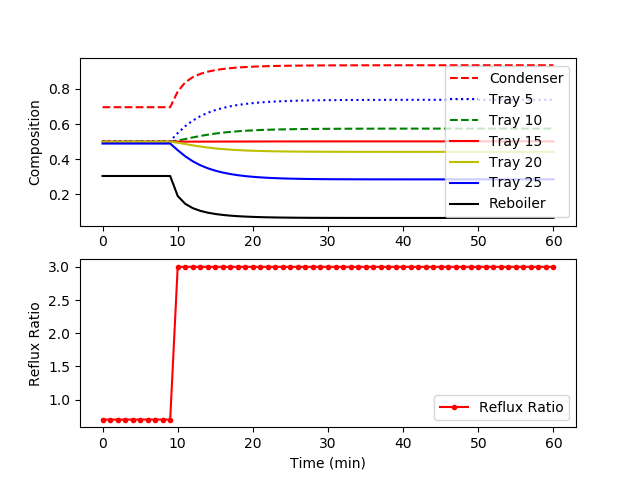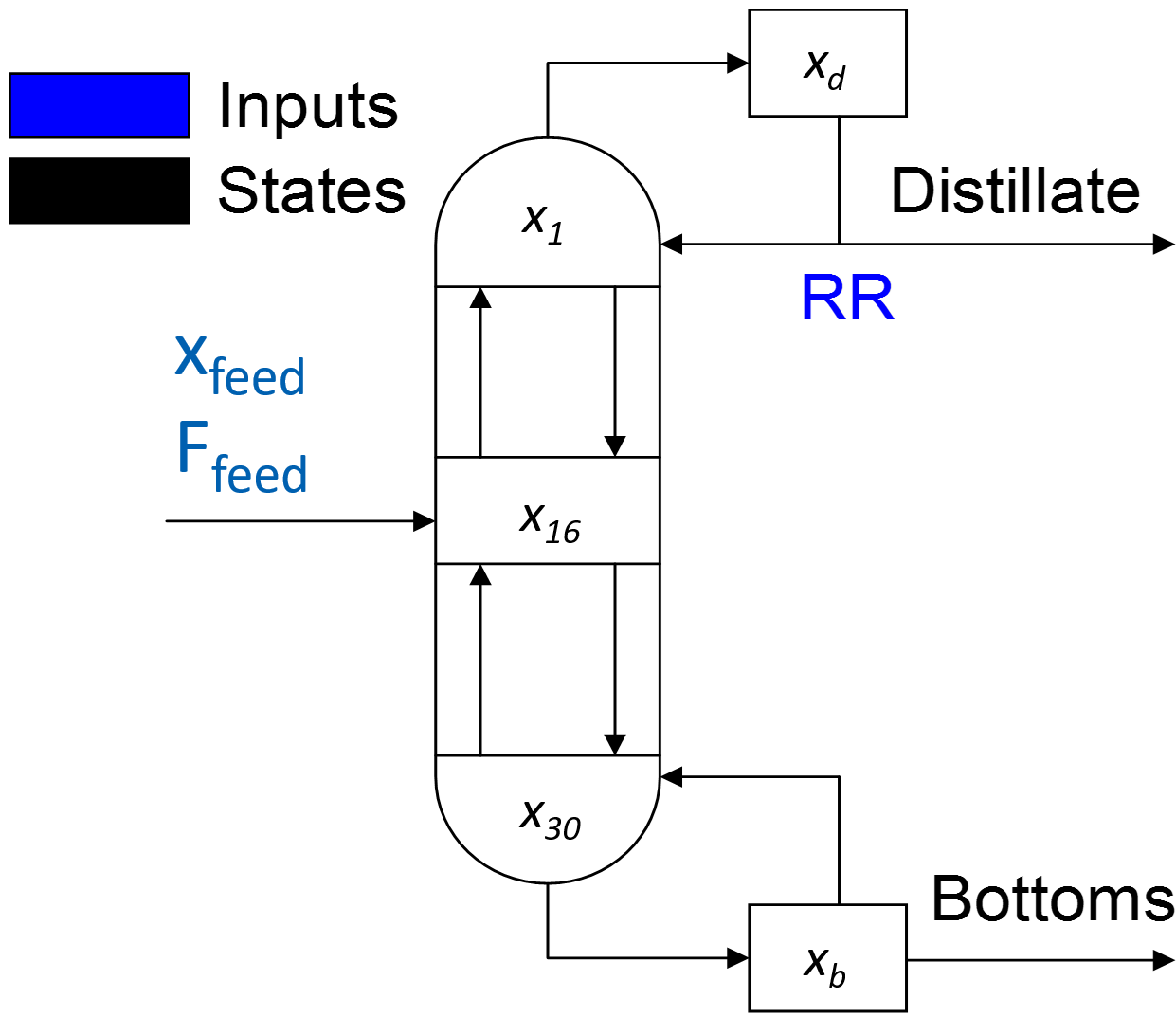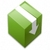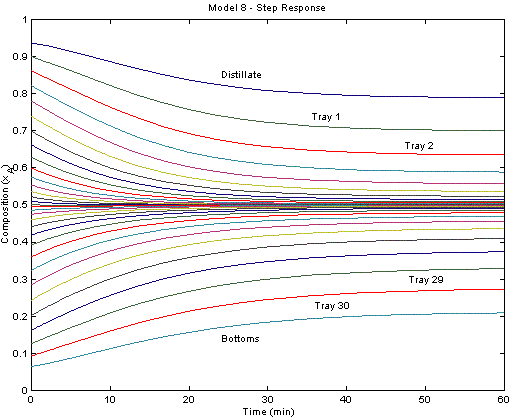Apps

## Apps.DistillationColumn History

March 17, 2019, at 09:17 PM by 45.56.3.173 -
Changed line 142 from:

(:html:)<font size=2><pre>

to:

(:source lang=python:)

Changed lines 248-250 from:

</pre></font> (:htmlend:)

to:

(:sourceend:)

February 12, 2019, at 02:20 PM by 10.37.233.114 -
Deleted lines 4-13:

### Multi-component Distillation Column

The distillation column in this example is built from a number of pre-existing model objects. The model objects used in this example include the distillation stage, feed, flash, mixer, splitter, stream lag, and vessel. These basic models are connected to form the multicomponent distillation tower.

Changed lines 250-259 from:

(:htmlend:)

to:

(:htmlend:)

### Multi-component Distillation Column

The distillation column in this example is built from a number of pre-existing model objects. The model objects used in this example include the distillation stage, feed, flash, mixer, splitter, stream lag, and vessel. These basic models are connected to form the multicomponent distillation tower.

February 12, 2019, at 02:19 PM by 10.37.233.114 -
Changed lines 27-146 from:

The figure below displays the system response after a step change in the reflux ratio from 3.0 to 1.5. Each trajectory represents the mole fraction of cyclohexane at each tray. The top reflux material becomes less pure (more n-heptane) due to the increased draw from the top of the column.

to:

The model is available as a Python script with solution provided by GEKKO. The following figure shows a step change in the reflux ratio from 0.7 to 3.0.(:source lang=python:) from gekko import GEKKO import numpy as np import matplotlib.pyplot as plt

1. Initialize Model

m = GEKKO()

1. Define constants
2. Reflux Ratio

rr=m.Param(value=0.7)

1. Feed flowrate (mol/min)

Feed=m.Const(value=2)

1. Mole fraction of feed

x_Feed=m.Const(value=.5)

1. Relative volatility = (yA/xA)/(yB/xB) = KA/KB = alpha(A,B)

vol=m.Const(value=1.6)

1. Total molar holdup on each tray

atray=m.Const(value=.25)

1. Total molar holdup in condenser

acond=m.Const(value=.5)

1. Total molar holdup in reboiler

areb=m.Const(value=.1)

1. mole fraction of component A

x=[] for i in range(32):

    x.append(m.Var(.3))

1. Define intermediates
2. Distillate flowrate (mol/min)

D=m.Intermediate(.5*Feed)

1. Liquid flowrate in rectification section (mol/min)

L=m.Intermediate(rr*D)

1. Vapor Flowrate in column (mol/min)

V=m.Intermediate(L+D)

1. Liquid flowrate in stripping section (mol/min)

FL=m.Intermediate(Feed+L)

1. vapor mole fraction of Component A
2. From the equilibrium assumption and mole balances
3. 1) vol = (yA/xA) / (yB/xB)
4. 2) xA + xB = 1
5. 3) yA + yB = 1

y=[] for i in range(32):

    y.append(m.Intermediate(x[i]*vol/(1+(vol-1)*x[i])))

1. condenser

m.Equation(acond*x.dt()==V*(y-x))

1. 15 column stages

n=1 for i in range(15):

    m.Equation(atray * x[n].dt() ==L*(x[n-1]-x[n]) - V*(y[n]-y[n+1]))
n=n+1

1. feed tray

m.Equation(atray * x.dt() == Feed*x_Feed + L*x - FL*x - V*(y-y))

1. 14 column stages

n=17 for i in range(14):

    m.Equation(atray * x[n].dt() == FL*(x[n-1]-x[n]) - V*(y[n]-y[n+1]))
n=n+1

1. reboiler

m.Equation(areb * x.dt() == FL*x - (Feed-D)*x - V*y)

1. steady state solution

m.solve() print(x) # with RR=0.7

1. switch to dynamic simulation

m.options.imode=4 nt = 61 m.time=np.linspace(0,60,61)

1. step change in reflux ratio

rr_step = np.ones(nt) * 0.7 rr_step[10:] = 3.0 rr.value=rr_step m.solve()

plt.subplot(2,1,1) plt.plot(m.time,x.value,'r--',label='Condenser') plt.plot(m.time,x.value,'b:',label='Tray 5') plt.plot(m.time,x.value,'g--',label='Tray 10') plt.plot(m.time,x.value,'r-',label='Tray 15') plt.plot(m.time,x.value,'y-',label='Tray 20') plt.plot(m.time,x.value,'b-',label='Tray 25') plt.plot(m.time,x.value,'k-',label='Reboiler') plt.ylabel('Composition') plt.legend(loc='best')

plt.subplot(2,1,2) plt.plot(m.time,rr.value,'r.-',label='Reflux Ratio') plt.ylabel('Reflux Ratio') plt.legend(loc='best')

plt.xlabel('Time (min)') plt.show() (:sourceend:)

The model is also available in the APMonitor Modeling Language. The figure below displays the system response after a step change in the reflux ratio from 3.0 to 1.5. Each trajectory represents the mole fraction of cyclohexane at each tray. The top reflux material becomes less pure (more n-heptane) due to the increased draw from the top of the column.

April 24, 2018, at 11:01 PM by 10.5.113.102 -

April 24, 2018, at 11:01 PM by 10.5.113.102 -
Changed lines 1-5 from:

## Distillation Columnsto:

(:html:) <iframe width="560" height="315" src="https://www.youtube.com/embed/a6eIEeCrJdU" frameborder="0" allow="autoplay; encrypted-media" allowfullscreen></iframe> (:htmlend:)

April 24, 2018, at 10:57 PM by 10.5.113.102 -December 22, 2011, at 06:50 AM by 69.169.188.228 -
Changed line 16 from:
to:
December 22, 2011, at 06:09 AM by 69.169.188.228 -
Changed line 16 from:Distillation Column PID and NLC control comparison Δ
to:
December 06, 2011, at 06:13 AM by 69.169.188.228 -
Changed lines 14-18 from:

This distillation column is a separation of cyclohexane and n-heptane. The two components are separated over 30 theoretical trays. In general, distillation column models are generally good test cases for nonlinear model reduction and identification. The concentrations at each stage or tray are highly correlated. The dynamics of the distillation process can be described by a relatively few number of underlying dynamic states. This model was published in:

to:

This distillation column is a separation of cyclohexane and n-heptane. The two components are separated over 30 theoretical trays. In general, distillation column models are generally good test cases for nonlinear model reduction and identification. The concentrations at each stage or tray are highly correlated. The dynamics of the distillation process can be described by a relatively few number of underlying dynamic states.Distillation Column PID and NLC control comparison Δ

This model was published in:

May 26, 2010, at 12:01 PM by 158.35.225.240 -
Changed lines 9-10 from:
to:
• (:html:)<a href="/online/view_pass.php?f=distill.apm">Solve Model Online Through a Web-based Interface</a>(:htmlend:)
May 26, 2010, at 11:58 AM by 158.35.225.240 -
Changed line 9 from:
• Solve model online
to:
March 06, 2010, at 09:39 AM by 206.180.155.75 -
Changed line 25 from:

(:html:)<font size=1><pre>

to:

(:html:)<font size=2><pre>

Changed lines 31-32 from:

to:

# Engineering, 26, pp. 1379-1397, (2002)

February 23, 2010, at 02:27 AM by 206.180.155.75 -
Changed lines 9-10 from:
• [https://apmonitor.ath.cx/online/view_pass.php?f=distill.apm| Solve model online]
to:
• Solve model online
February 23, 2010, at 02:25 AM by 206.180.155.75 -
• [https://apmonitor.ath.cx/online/view_pass.php?f=distill.apm| Solve model online]
April 23, 2009, at 04:29 PM by 158.35.225.231 -

# Reboiler 0.06458317697321

Model binary

  Parameters
! reflux ratio
rr = 0.7

! Feed Flowrate (mol/min)
Feed =  2.0 ! 24.0/60.0
! Mole Fraction of Feed
x_Feed = 0.5
! Relative Volatility = (yA/xA)/(yB/xB) = KA/KB = alpha(A,B)
vol=1.6
! Total Molar Holdup in the Condenser
atray=0.25
! Total Molar Holdup on each Tray
acond=0.5
! Total Molar Holdup in the Reboiler
areb=0.1
End Parameters

Variables
! mole fraction of component A
x[1:32] = 0.3
End Variables

Intermediates
! Distillate Flowrate (mol/min)
D=0.5*Feed
! Flowrate of the Liquid in the Rectification Section (mol/min)
L=rr*D
! Vapor Flowrate in the Column (mol/min)
V=L+D
! Flowrate of the Liquid in the Stripping Section (mol/min)
FL=Feed+L

! Vapor Mole Fractions of Component A
! From the equilibrium assumption and mole balances
! 1) vol = (yA/xA) / (yB/xB)
! 2) xA + xB = 1
! 3) yA + yB = 1
y[1:32] = x[1:32]*vol/(1+(vol-1)*x[1:32])
End Intermediates

Equations
! condenser
acond * $x = V*(y-x) ! 15 column stages atray *$x[2:16]  = L*(x[1:15]-x[2:16]) - V*(y[2:16]-y[3:17])

! feed tray
atray * $x = Feed*x_Feed + L*x - FL*x - V*(y-y) ! 14 column stages atray *$x[18:31] = FL*(x[17:30]-x[18:31]) - V*(y[18:31]-y[19:32])

! reboiler
areb  * \$x = FL*x - (Feed-D)*x - V*y
End Equations


End Model

April 23, 2009, at 04:29 PM by 158.35.225.231 -
Changed lines 21-28 from:to:(:html:)<font size=1><pre>

</pre></font> (:htmlend:)

November 04, 2008, at 10:44 PM by 158.35.225.231 -
Changed line 8 from:
to:
November 04, 2008, at 10:44 PM by 158.35.225.231 -
Changed line 13 from:

This distillation column is a separation of cyclohexane and n-heptane. The two components are separated over 30 theoretical trays. In general, distillation column models are generally good test cases for nonlinear model reduction and identification. The concentrations at each stage or tray are highly correlated. The dynamics of the distillation process can be described by a relatively few number of underlying dynamic states. This model was published in

to:

This distillation column is a separation of cyclohexane and n-heptane. The two components are separated over 30 theoretical trays. In general, distillation column models are generally good test cases for nonlinear model reduction and identification. The concentrations at each stage or tray are highly correlated. The dynamics of the distillation process can be described by a relatively few number of underlying dynamic states. This model was published in:

November 04, 2008, at 10:44 PM by 158.35.225.231 -

### Multi-component Distillation Column

Changed lines 11-14 from:

Binary Distillation Column with 30 trays (cyclohexane n-heptane)

Distillation column models are generally good test cases for nonlinear model reduction and identification. The concentrations at each stage or tray are highly correlated. The dynamics of the distillation process can be described by a relatively few number of underlying dynamic states. A couple papers have been published with this model as an example application. One in particular is:

to:

### Binary Distillation Column

This distillation column is a separation of cyclohexane and n-heptane. The two components are separated over 30 theoretical trays. In general, distillation column models are generally good test cases for nonlinear model reduction and identification. The concentrations at each stage or tray are highly correlated. The dynamics of the distillation process can be described by a relatively few number of underlying dynamic states. This model was published in

November 04, 2008, at 10:35 PM by 158.35.225.231 -

The distillation column in this example is built from a number of pre-existing model objects. The model objects used in this example include the distillation stage, feed, flash, mixer, splitter, stream lag, and vessel. These basic models are connected to form the multicomponent distillation tower.

Binary Distillation Column with 30 trays (cyclohexane n-heptane)

Distillation column models are generally good test cases for nonlinear model reduction and identification. The concentrations at each stage or tray are highly correlated. The dynamics of the distillation process can be described by a relatively few number of underlying dynamic states. A couple papers have been published with this model as an example application. One in particular is:

Hahn, J. and T.F. Edgar, An improved method for nonlinear model reduction using balancing of empirical gramians, Computers and Chemical Engineering, 26, pp. 1379-1397, (2002)

The figure below displays the system response after a step change in the reflux ratio from 3.0 to 1.5. Each trajectory represents the mole fraction of cyclohexane at each tray. The top reflux material becomes less pure (more n-heptane) due to the increased draw from the top of the column.

November 04, 2008, at 10:28 PM by 158.35.225.231 -
Changed lines 1-5 from:

to:

## Distillation Columns